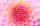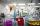# Solve equation

solve equation:Result

a1 =  0
a2 =  0

#### Solution:Leave us a comment of example and its solution (i.e. if it is still somewhat unclear...):

Showing 0 comments:Be the first to comment!#### To solve this example are needed these knowledge from mathematics:

Looking for help with calculating roots of a quadratic equation? Do you have a linear equation or system of equations and looking for its solution? Or do you have quadratic equation?

## Next similar examples:

1. Completing squareSolve the quadratic equation: m2=4m+20 using completing the square method
2. Solve 3Solve quadratic equation: (6n+1) (4n-1) = 3n2
3. Variation equationSolve combinatorics equation: V(2, x+8)=72
4. Reciprocal equation 2Solve this equation: x + 5/x - 6 = 4/11
5. ProductThe product of two consecutive odd numbers is 8463. What are this numbers?
6. Square root 2If the square root of 3m2 +22 and -x = 0, and x=7, what is m?
7. EquationEquation ? has one root x1 = 8. Determine the coefficient b and the second root x2.
8. RootsDetermine the quadratic equation absolute coefficient q, that the equation has a real double root and the root x calculate: ?
9. Cinema 4In cinema are 1656 seats and in the last row are 105 seats , in each next row 3 seats less. How many are the total rows in cinema?
10. Quadratic equationFind the roots of the quadratic equation: 3x2-4x + (-4) = 0.
11. Evaluation of expressionsIf a2-3a+1=0, find (i)a2+1/a2 (ii) a3+1/a3
12. DiscriminantDetermine the discriminant of the equation: ?
13. VariableFind variable P: PP plus P x P plus P = 160
14. Difference AP 4Calculate the difference of the AP if a1 = 0.5, a2 + a3 = -1.1
15. Crystal waterThe chemist wanted to check the content of water of crystallization of chromic potassium alum K2SO4 * Cr2 (SO4) 3 * 24 H2O, which was a long time in the laboratory. From 96.8 g of K2SO4 * Cr2 (SO4) 3 * 24 H2O prepared 979 cm3 solution of base. S
16. CalculationHow much is sum of square root of six and the square root of 225?
17. Theorem proveWe want to prove the sentense: If the natural number n is divisible by six, then n is divisible by three. From what assumption we started?